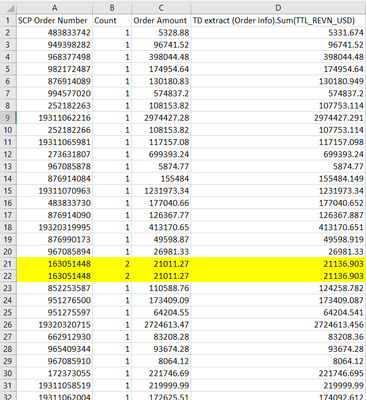cancel
Showing results for
Did you mean:Helper II

## Sumif with condition

Hi everybody,

I have the following problem in Power Query. I would need to create a function that would add the values ​​in the Order Amount column and divide them by the value in the TD exract column (Order info). Sum ... but the addition of these two numbers will only be done if the Count column has a value of 2 or 3. So, for example, in the highlighted example on lines 21 and 22, I would need to find the value 2 in the Count column, see if the SCP Order Number column is the same number, and if that number is the same, add up their values ​​in the Order Amount column and divide them by one of the values ​​(it doesn't matter which one is the same) in column D. The result would therefore be: (21011.27 + 21011.27) /21136.903 It would be best if only one record (one SCP Order Number) is created in the end and a given calculation is created in a new column.Can anyone help me? Thank you very much

1 ACCEPTED SOLUTIONCommunity Support

Use the Group by feature under Transform tab. Then select the Sum Order Amount column and divide it by TD extract Sum column.``````let
Source = Table.FromRows(Json.Document(Binary.Decompress(Binary.FromText("lYzBCQAhDAR7yVskuzEaaxH7b+OMcHDfewwM7LBrCbqpo7WQIkygQOW4Cut1qskuP8Jw0s0jFyRQj6ijp7PRjwdvOh0c3VXfdFjTWXV+/Pzs/QA=", BinaryEncoding.Base64), Compression.Deflate)), let _t = ((type nullable text) meta [Serialized.Text = true]) in type table [#"SCP Order Number" = _t, Count = _t, #"Order Amount" = _t, #"TD extract (Order info).Sum" = _t]),
#"Changed Type" = Table.TransformColumnTypes(Source,{{"SCP Order Number", Int64.Type}, {"Count", Int64.Type}, {"Order Amount", type number}, {"TD extract (Order info).Sum", type number}}),
#"Grouped Rows" = Table.Group(#"Changed Type", {"SCP Order Number", "Count"}, {{"Sum Order Amount", each List.Sum([Order Amount]), type nullable number}, {"TD extract Sum", each List.Max([#"TD extract (Order info).Sum"]), type nullable number}}),
#"Inserted Division" = Table.AddColumn(#"Grouped Rows", "Division", each [Sum Order Amount] / [TD extract Sum], type number)
in
#"Inserted Division"``````

Best Regards,
Community Support Team _ Jing
If this post helps, please Accept it as Solution to help other members find it.

3 REPLIES 3Community Support

Use the Group by feature under Transform tab. Then select the Sum Order Amount column and divide it by TD extract Sum column.``````let
Source = Table.FromRows(Json.Document(Binary.Decompress(Binary.FromText("lYzBCQAhDAR7yVskuzEaaxH7b+OMcHDfewwM7LBrCbqpo7WQIkygQOW4Cut1qskuP8Jw0s0jFyRQj6ijp7PRjwdvOh0c3VXfdFjTWXV+/Pzs/QA=", BinaryEncoding.Base64), Compression.Deflate)), let _t = ((type nullable text) meta [Serialized.Text = true]) in type table [#"SCP Order Number" = _t, Count = _t, #"Order Amount" = _t, #"TD extract (Order info).Sum" = _t]),
#"Changed Type" = Table.TransformColumnTypes(Source,{{"SCP Order Number", Int64.Type}, {"Count", Int64.Type}, {"Order Amount", type number}, {"TD extract (Order info).Sum", type number}}),
#"Grouped Rows" = Table.Group(#"Changed Type", {"SCP Order Number", "Count"}, {{"Sum Order Amount", each List.Sum([Order Amount]), type nullable number}, {"TD extract Sum", each List.Max([#"TD extract (Order info).Sum"]), type nullable number}}),
#"Inserted Division" = Table.AddColumn(#"Grouped Rows", "Division", each [Sum Order Amount] / [TD extract Sum], type number)
in
#"Inserted Division"``````

Best Regards,
Community Support Team _ Jing
If this post helps, please Accept it as Solution to help other members find it.Helper II

Thank u very much. It works! 🙂Super User

In Power Query group your data by the first column, aggregate the second column as sum and the third column as max.